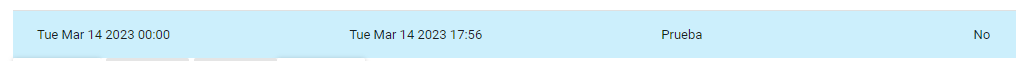# DX Unified Infrastructure Management

View Only

## Problem recalculating SLAs#### Oswaldo Muñoz13 days ago#### Olaf Pape10 days ago#### Oswaldo Muñoz10 days ago• #### 1.  Problem recalculating SLAs

Posted 13 days ago

Hello,

We have a problem when we recalculate an SLA. When the period is all day, the recalculation is done well, but when we recalculate only a few hours of the day, the recalculation does not work.

For example:

This is the excluded periodand this is the recalculateThis example doesn´t work, but if the excluded period is the whole day, it does work.

• #### 2.  RE: Problem recalculating SLAs

Posted 10 days ago

Hi,

which version of the sla_engine do you run?

• #### 3.  RE: Problem recalculating SLAs

Posted 10 days ago

Hello,

The SLA engine version is 20.11.

Thnaks.

• #### 4.  RE: Problem recalculating SLAs

Posted 10 days ago

Hi,

20.11 is really old. Since we have seen a similar problem with 20.41, it might already exist with 20.11.

As soon as you use Office Hours in the SLA, it happens that the calculation uses wrong time periods. If I remember correctly, the calculation periods are offset by one day. With a higher log level (I guess loglevel=4) you can see this nicely in the logfile (but unfortunately you have to convert EPOCH timestamps).